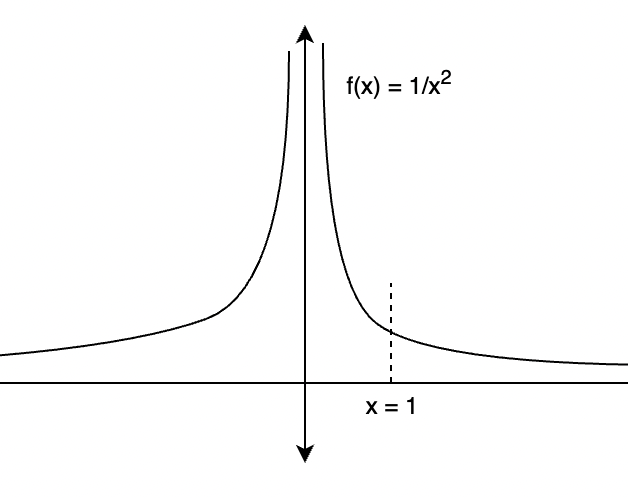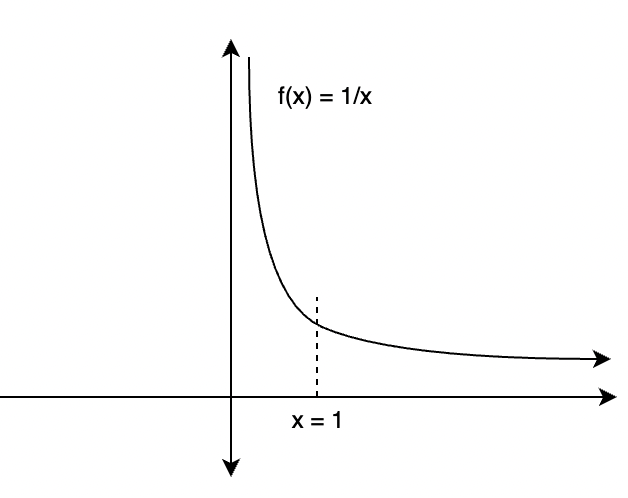Open in App
Not now

# Improper Integrals

• Last Updated : 30 Jun, 2021

Geometrically speaking, integrals are the way to compute the area or volume under curves. These methods allow mathematicians to compute the area under arbitrarily complex curves. These types of integrals are called definite integrals. Definite integrals are built upon the idea of indefinite integrals. These indefinite integrals are nothing but anti-derivatives of the functions. Anti-derivatives are the reverse of differentiation. Let’s see these ideas in detail.

### What are Improper Integrals?

Improper integrals are definite integrals where one or both of the boundaries are at infinity or where the Integrand has a vertical asymptote in the interval of integration. Computing the area up to infinity seems like an intractable problem, but through some clever manipulation, such problems can be solved. Let’s consider a function f(x), then the area under the curve enclosed by x-axis between the limits x = a and x = b is denoted as,Since both limits here are finite, this is called a proper integral. A proper integral with one infinite bound will be denoted as,orConsider an example to get a better understanding.

Example: Compute the following definite integral.Solution:

The graph of this function is shown the figure below. The goal is to calculate the mentioned area. Notice that the area is not diverging as the function is going towards zero asymptotically.This can be re-written as,Now this is just a definite integral, to solve this second part of fundamental theorem of calculus can be used.Sometimes integrals have both their limits bounds as infinity. Such integrals are called improper integrals with two infinite boundsor### Divergent Improper Integral

In the previous function, the limit of the area calculated up to infinity is finite. But often in some cases, the integrals do not converge to a finite value. Intuitively, this means that the area enclosed under the curve is not finite. Consider an example for this type of case to get a better understanding of such integrals.

Example: Compute the following integral.Solution:

The graph of this function is given below.Let’s compute the area under this curve using the same method as above.Rewriting the given integral.Now this is again just a definite integral, to solve this second part of fundamental theorem of calculus can be used.Since this limit diverges. The area under the curve is infinite.

Let’s see some problems on these concepts.

### Sample Problems

Question 1: Compute the following definite integral.Solution:This can be re-written as,Now this is just a definite integral, to solve this second part of the fundamental theorem of calculus can be used.Question 2: Compute the following definite integral.Solution:This can be re-written as,Now this is just a definite integral, to solve this second part of fundamental theorem of calculus can be used.This limit evaluates to infinity. Thus, the area under the curve is infinite.

Question 3: Compute the following definite integral.Solution:This can be re-written as,Now this is just a definite integral, to solve this second part of the fundamental theorem of calculus can be used.This limit evaluates to infinity. Thus, the area under the curve is infinite.

Question 4: Compute the following definite integral.Solution:This can be re-written as,Now this is just a definite integral, to solve this second part of fundamental theorem of calculus can be used.This area cannot be calculated. This is infinite.

Question 5: Compute the following definite integral.Solution:This can be re-written as,Now this is just a definite integral, to solve this second part of fundamental theorem of calculus can be used.This area cannot be calculated. This is infinite.

Question 6: Determine whether this improper integral is convergent or divergent.Solution:This can be re-written as,Now this is just a definite integral, to solve this second part of fundamental theorem of calculus can be used.The limit is not defined, thus this integral is divergent.

Question 7: Determine whether this improper integral is convergent or divergent.Solution:This can be re-written as,Now this is just a definite integral, to solve this second part of fundamental theorem of calculus can be used.My Personal Notes arrow_drop_up